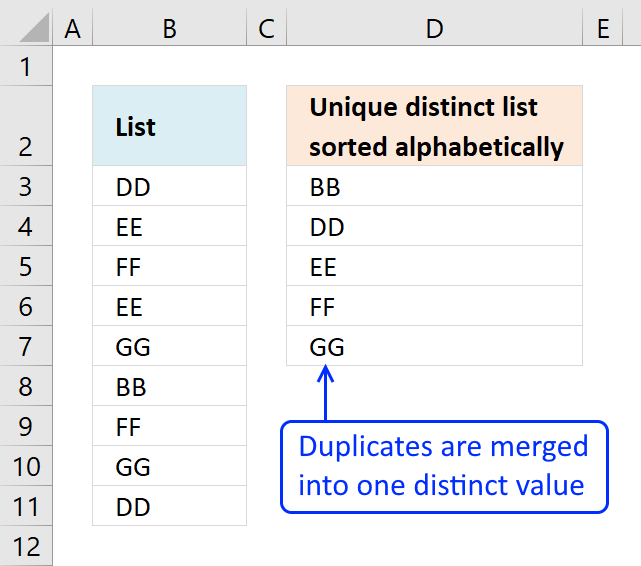Author: Oscar Cronquist Article last updated on February 11, 2018The array formula in cell D3 extracts unique distinct values sorted A to Z, from column B to column D. Unique distinct values are all values except duplicates.

Example, in column B value "DD" exists twice in cell B3 and B11. In column D value "DD" exists only once since it is a unique distinct list.

Array formula in cell D3:

=INDEX(\$B\$3:\$B\$11, MATCH(SMALL(IF(COUNTIF(\$D\$2:D2, \$B\$3:\$B\$11)=0, COUNTIF(\$B\$3:\$B\$11, "<"&\$B\$3:\$B\$11), ""), 1), COUNTIF(\$B\$3:\$B\$11, "<"&\$B\$3:\$B\$11), 0))

Watch a video that explains how to use it and how it works:

Learn how to filter values with a condition and return unique distinct values sorted from A to Z:

Unique distinct list sorted alphabetically based on a condition

The array formula in cell E6 filters values in column C based on value in cell E3, the output is […]

How to create an array formula

1. Double click on cell D3
2. Copy (Ctrl +c) amd paste (Ctrl+v) above formula to cell D3
3. Press and hold Ctrl + Shift simultaneously
4. Press Enter once
5. Release all keys

The formula in the formula bar should now look like this: {=formula}
Don't enter the curly brackets yourself, they appear automatically.How to enter an array formula

Array formulas allows you to do advanced calculations not possible with regular formulas.

How to copy array formula

1. Select cell D3
2. Copy cell  (Keyboard shortcut: Ctrl + c)
3. Select cell range D4:D7
4. Paste (Keyboard shortcut: Ctrl + v)

Explaining array formula in cell

Step 1 - Identify values not yet shown above current cell

The COUNTIF function counts the number of times a value exists in a cell range.

Cell range \$D\$2:D2 changes as the formula is copied down to cells below. This makes it possible to avoid duplicate values in the list.

COUNTIF(\$D\$2:D2,\$B\$3:\$B\$11)=0

becomes

COUNTIF("Unique list sorted alphabetically",{"DD";"EE";"FF";"EE";"GG";"BB";"FF";"GG";"DD"})=0

becomes

{0;0;0;0;0;0;0;0;0}=0

and returns

{TRUE; TRUE; TRUE; TRUE; TRUE; TRUE; TRUE; TRUE; TRUE}

Step 2 - Create an array with a ranking sort number

COUNTIF(\$B\$3:\$B\$11,"<"&\$B\$3:\$B\$11)

becomes

{1;3;5;3;7;0;5;7;1}

Step 3 - Convert array with not displayed values to an array containing rank numbers

The IF function converts not yet displayed values into alphabeically ranked numbers.

IF(COUNTIF(\$D\$2:D2,\$B\$3:\$B\$11)=0,COUNTIF(\$B\$3:\$B\$11,"<"&\$B\$3:\$B\$11),"")

becomes

IF({TRUE; TRUE; TRUE; TRUE; TRUE; TRUE; TRUE; TRUE; TRUE},{1;3;5;3;7;0;5;7;1},"")

and returns

{1;3;5;3;7;0;5;7;1}

Step 4 - Find the smallest value in array

The SMALL function extracts the smallest number in the array.

SMALL(IF(COUNTIF(\$D\$2:D2, \$B\$3:\$B\$11)=0, COUNTIF(\$B\$3:\$B\$11,"<"&\$B\$3:\$B\$11), ""),1)

becomes

SMALL({1;3;5;3;7;0;5;7;1},1)

and returns 0.

How to use the SMALL function

The SMALL function lets you extract a number in a cell range based on how small it is compared to the other numbers in the group.

Step 5 - Find relative position in the array

The MATCH function finds the position of the next alphabetically sorted value.

MATCH(SMALL(IF(COUNTIF(\$D\$2:D2, \$B\$3:\$B\$11)=0, COUNTIF(\$B\$3:\$B\$11,"<"&\$B\$3:\$B\$11),""), 1),COUNTIF(\$B\$3:\$B\$11, "<"&\$B\$3:\$B\$11), 0)

becomes

MATCH(0,COUNTIF(\$B\$3:\$B\$11, "<"&\$B\$3:\$B\$11), 0)

becomes

MATCH(0,{1;3;5;3;7;0;5;7;1}, 0)

and returns 6.

Step 6 - Return value in data based on row coordinate

The INDEX function returns a value based on row and column number.

INDEX(\$B\$3:\$B\$11, MATCH(SMALL(IF(COUNTIF(\$D\$2:D2, \$B\$3:\$B\$11)=0, COUNTIF(\$B\$3:\$B\$11, "<"&\$B\$3:\$B\$11), ""), 1), COUNTIF(\$B\$3:\$B\$11, "<"&\$B\$3:\$B\$11), 0))

becomes

INDEX(\$B\$3:\$B\$11, 6)

becomes

INDEX({"DD"; "EE"; "FF"; "EE"; "GG"; "BB"; "FF"; "GG"; "DD"}, 6)

and returns BB in cell D3.